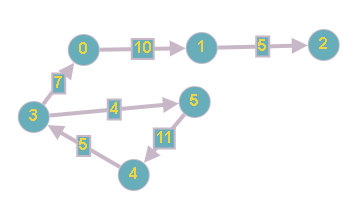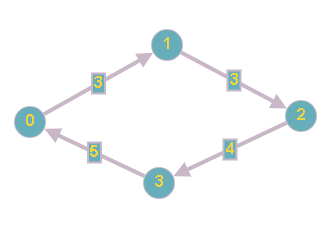1

# Boat Journey

Difficulty: HARDContributed By
Avg. time to solve
60 min
Success Rate
40%

Problem Statement

#### Note : Energy consumption of one journey is equal to the sum of energy of each trip. There can be more than one river between any two cities.

##### Input Format:
``````The first line contains one positive integer ‘T’, denoting the number of test cases, then ‘T’ test cases follows

The first line of each test case contains three integers ‘N’  ‘M’ and ‘Q’, denoting the number of cities, the number of rivers and number of travel.

The next ‘M’ lines of each test case contains three space-separated integers ’u’ , ‘v’ and ‘w’, denoting connection between cities ‘u’ and ‘v’ where river flow from ‘u’ to ‘v’ and energy required against flow is ‘w’.

The next ‘Q’ lines of each test case contain two integers ‘x’ and ‘y’, denoting each travel from ‘x’ to ‘y’.
``````
##### Output Format:
``````The first and the only line of each test case contains one integer ‘X’, denoting the minimum energy required to complete his journey using his special power ( he may not use his special power).

Output of each test case will be printed on a separate line.
``````
##### Note:
``````You do not need to print anything, it has already been taken care of. Just implement the given function.
``````
##### Constraints:
``````1 <= T <= 5
1 <= N, M, Q <= 1000
0 <= u, v <= N - 1, u != v
0 <= ‘x’, ‘y’ <= N - 1

Time Limit: 1 sec.
``````
##### Sample Input 1:
``````2
6 6 2
0 1 10
1 2 5
3 0 7
3 5 4
5 4 11
4 3 5
4 0
1 5
4 4 2
0 1 3
1 2 3
2 3 4
3 0 5
0 2
2 0
``````
##### Sample Output 1:
``````7
0
``````
##### Explanation of Sample Output1:
``````In the first test case, the Starlink Kingdom looks like this:
```````````` We can change the direction of the river flow between 0 and 1.
On the first trip we can go from city 4 to 0 without using any energy.
On the second trip we swam against the river 0 - 3. So the minimum energy required is 7.

In the second test case, the Starlink Kingdom looks like this:
````````````We can change the direction of the river flow between (0 4) and (4 3).
On the first trip we can go from city 0 -> 1 -> 3 without using any energy.
On the second trip we can go from city 3 -> 4 -> 0  without using any energy.
So the total required is 0.
``````
##### Sample Input 2:
``````2
2 2 2
0 1 10
1 0 10
0 1
1 0
3 3 2
1 2 10
1 2 15
0 1 10
2 0
0 2
``````
##### Sample Output 2:
``````0
10
``````Console